Circular Motion and Satellite Motion - Lesson 2 - Applications of Circular Motion

# Newton's Second Law - Revisited

Newton's second law states that the acceleration of an object is directly proportional to the net force acting upon the object and inversely proportional to the mass of the object. The law is often expressed in the form of the following two equations.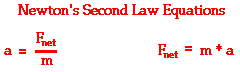### Newton's Second Law and a Force Analysis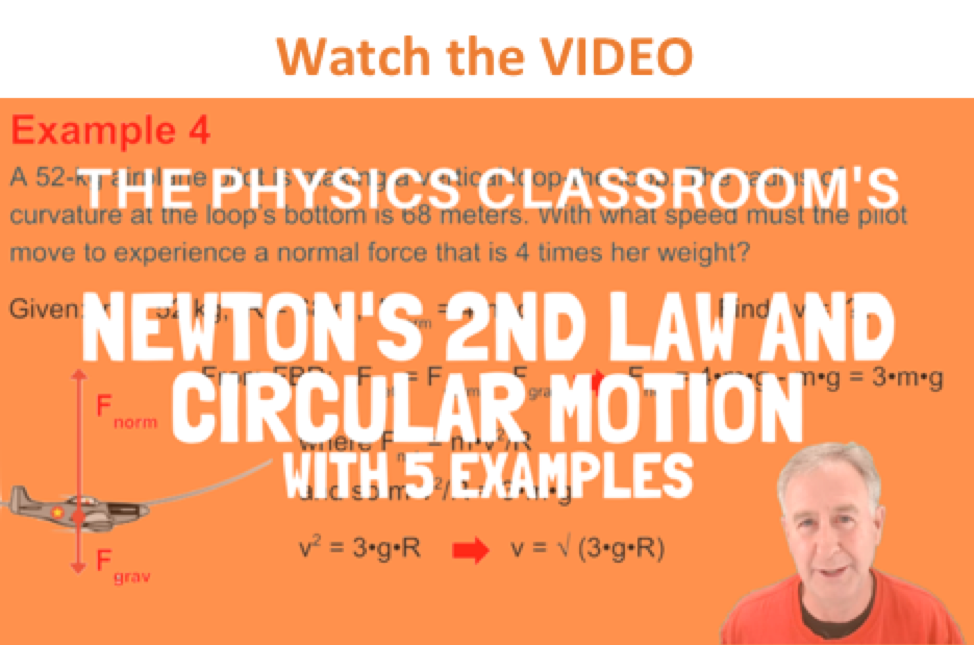In Unit 2 of The Physics Classroom, Newton's second law was used to analyze a variety of physical situations. The idea was that if any given physical situation is analyzed in terms of the individual forces that are acting upon an object, then those individual forces must add up as vectors to the net force. Furthermore, the net force must be equal to the mass times the acceleration. Subsequently, the acceleration of an object can be found if the mass of the object and the magnitudes and directions of each individual force are known. And the magnitude of any individual force can be determined if the mass of the object, the acceleration of the object, and the magnitude of the other individual forces are known. The process of analyzing such physical situations in order to determine unknown information is dependent upon the ability to represent the physical situation by means of a free-body diagram. A free-body diagram is a vector diagram that depicts the relative magnitude and direction of all the individual forces that are acting upon the object.

 Review of Unit 2 Such force analyses were presented in Unit 2 and elaborately discussed. Perhaps you would wish to review these lessons before proceeding through the remainder of Unit 6. If so, use the following links to Unit 2 sub-lessons.

In this Lesson, we will use Unit 2 principles (free-body diagrams, Newton's second law equation, etc.) and circular motion concepts in order to analyze a variety of physical situations involving the motion of objects in circles or along curved paths. The mathematical equations discussed in Lesson 1 and the concept of a centripetal force requirement will be applied in order to analyze roller coasters and other amusement park rides and various athletic movements.

### CIrcular Motion in a Horizontal Plane

To illustrate how circular motion principles can be combined with Newton's second law to analyze a physical situation, consider a car moving in a horizontal circle on a level surface. The diagram below depicts the car on the left side of the circle.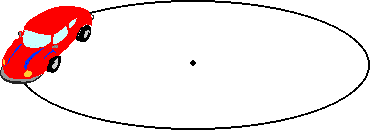Applying the concept of a centripetal force requirement, we know that the net force acting upon the object is directed inwards. Since the car is positioned on the left side of the circle, the net force is directed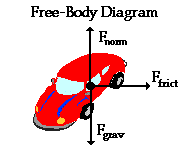rightward. An analysis of the situation would reveal that there are three forces acting upon the object - the force of gravity (acting downwards), the normal force of the pavement (acting upwards), and the force of friction (acting inwards or rightwards). It is the friction force that supplies the centripetal force requirement for the car to move in a horizontal circle. Without friction, the car would turn its wheels but would not move in a circle (as is the case on an icy surface). This analysis leads to the free-body diagram shown at the right. Observe that each force is represented by a vector arrow that points in the specific direction that the force acts; also notice that each force is labeled according to type (Ffrict, Fnorm, and Fgrav). Such an analysis is the first step of any problem involving Newton's second law and a circular motion.

Now consider the following two problems pertaining to this physical scenario of the car making a turn on a horizontal surface.

 Sample Problem #1 The maximum speed with which a 945-kg car makes a 180-degree turn is 10.0 m/s. The radius of the circle through which the car is turning is 25.0 m. Determine the force of friction and the coefficient of friction acting upon the car. Sample Problem #2 The coefficient of friction acting upon a 945-kg car is 0.850. The car is making a 180-degree turn around a curve with a radius of 35.0 m. Determine the maximum speed with which the car can make the turn.

Sample problem #1 provides kinematic information (v and R) and requests the value of an individual force. As such the solution of the problem will demand that the acceleration and the net force first be determined; then the individual force value can be found by use of the free-body diagram. Sample problem #2 provides information about the individual force values (or at least information that allows for the determination of the individual force values) and requests the value of the maximum speed of the car. As such, its solution will demand that individual force values be used to determine the net force and acceleration; then the acceleration can be used to determine the maximum speed of the car. The two problems will be solved using the same general principles. Yet because the given and requested information is different in each, the solution method will be slightly different.

Solution to Sample Problem #1

The known information and requested information in sample problem #1 is:

 Known Information: m = 945 kg v = 10.0 m/s R = 25.0 m Requested Information: Ffrict = ??? μ = ???? (μ - coefficient of friction)

The mass of the object can be used to determine the force of gravity acting in the downward direction. Use the equation

Fgrav = m * g

where g is 9.8 m/s/s. Knowing that there is no vertical acceleration of the car, it can be concluded that the vertical forces balance each other. Thus, Fgrav = Fnorm= 9261 N. This allows us to determine two of the three forces identified in the free-body diagram. Only the friction force remains unknown.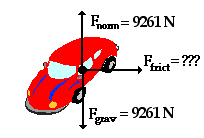Since the force of friction is the only horizontal force, it must be equal to the net force acting upon the object. So if the net force can be determined, then the friction force is known. To determine the net force, the mass and the kinematic information (speed and radius) must be substituted into the following equation: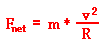Substituting the given values yields a net force of 3780 Newton. Thus, the force of friction is 3780 N.

Finally the coefficient of friction (μ) can be determined using the equation that relates the coefficient of friction to the force of friction and the normal force.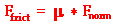Substituting 3780 N for Ffrict and 9261 N for Fnorm yields a coefficient of friction of 0.408.

Solution to Sample Problem #2

Once again, the problem begins by identifying the known and requested information. The known information and requested information in the sample problem #2 is:

 Known Information: m = 945 kg μ = 0.85 (coefficient of friction) R = 35.0 m Requested Information: v = ??? (the minimum speed would be the speed achieved with the given friction coefficient)

The mass of the car can be used to determine the force of gravity acting in the downward direction. Use the equation

Fgrav = m * g

where g is 9.8 m/s/s. Knowing that there is no vertical acceleration of the car, it can be concluded that the vertical forces balance each other. Thus, Fgrav = Fnorm= 9261 N. Since the coefficient of friction (μ) is given, the force of friction can be determined using the following equation:This allows us to determine all three forces identified in the free-body diagram.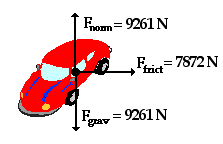The net force acting upon any object is the vector sum of all individual forces acting upon that object. So if all individual force values are known (as is the case here), the net force can be calculated. The vertical forces add to 0 N. Since the force of friction is the only horizontal force, it must be equal to the net force acting upon the object. Thus, Fnet = 7872 N.

Once the net force is determined, the acceleration can be quickly calculated using the following equation.

Fnet = m*a

Substituting the given values yields an acceleration of 8.33 m/s/s. Finally, the speed at which the car could travel around the turn can be calculated using the equation for centripetal acceleration: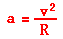Substituting the known values for a and R into this equation and solving algebraically yields a maximum speed of 17.1 m/s.

### Developing the Proper Problem-Solving Approach

Each of the two sample problems above was solved using the same basic problem-solving approach. The approach can be summarized as follows.

 Suggested Method of Solving Circular Motion Problems From the verbal description of the physical situation, construct a free-body diagram. Represent each force by a vector arrow and label the forces according to type. Identify the given and the unknown information (express in terms of variables such as m= , a= , v= , etc.). If any of the individual forces are directed at angles to the horizontal and vertical, then use vector principles to resolve such forces into horizontal and vertical components. Determine the magnitude of any known forces and label on the free-body diagram. (For example, if the mass is given, then the Fgrav can be determined. And as another example, if there is no vertical acceleration, then it is known that the vertical forces or force components balance, allowing for the possible determination of one or more of the individual forces in the vertical direction.) Use circular motion equations to determine any unknown information. (For example, if the speed and the radius are known, then the acceleration can be determined. And as another example, if the period and radius are known, then the acceleration can be determined.) Use the remaining information to solve for the requested information. If the problem requests the value of an individual force, then use the kinematic information (R, T and v) to determine the acceleration and the Fnet ; then use the free-body diagram to solve for the individual force value. If the problem requests the value of the speed or radius, then use the values of the individual forces to determine the net force and acceleration; then use the acceleration to determine the value of the speed or radius.

The method prescribed above will serve you well as you approach circular motion problems. However, one caution is in order. Every physics problem differs from the previous problem. As such, there is no magic formula for solving every one. Using an appropriate approach to solving such problems (which involves constructing a FBD, identifying known information, identifying the requested information, and using available equations) will never eliminate the need to think, analyze and problem-solve. For this reason, make an effort to develop an appropriate approach to every problem; yet always engage your critical analysis skills in the process of the solution. If physics problems were a mere matter of following a foolproof, 5-step formula or using some memorized algorithm, then we wouldn't call them "problems."

### We Would Like to Suggest ...Sometimes it isn't enough to just read about it. You have to interact with it! And that's exactly what you do when you use one of The Physics Classroom's Interactives. We would like to suggest that you combine the reading of this page with the use of our Uniform Circular Motion Interactive, our Roller Coaster Model Interactive, or our Horizontal Circle Simulation. You can find these Interactives in the Physics Interactives section of our website. These Interactives allow a learner to interactively explore the relationships between the velocity, acceleration, and force for an object moving in a circle or along a curved path.

Use your understanding of Newton's second law and circular motion principles to determine the unknown values in the following practice problems. Click the button to check your answers.

1. A 1.50-kg bucket of water is tied by a rope and whirled in a circle with a radius of 1.00 m. At the top of the circular loop, the speed of the bucket is 4.00 m/s. Determine the acceleration, the net force and the individual force values when the bucket is at the top of the circular loop.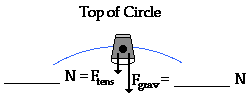m = 1.5 kg

a = ________ m/s/s

Fnet = _________ N

2. A 1.50-kg bucket of water is tied by a rope and whirled in a circle with a radius of 1.00 m. At the bottom of the circular loop, the speed of the bucket is 6.00 m/s. Determine the acceleration, the net force and the individual force values when the bucket is at the bottom of the circular loop.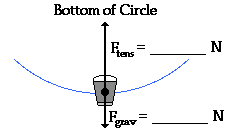m = 1.5 kg

a = ________ m/s/s

Fnet = _________ N

Next Section: